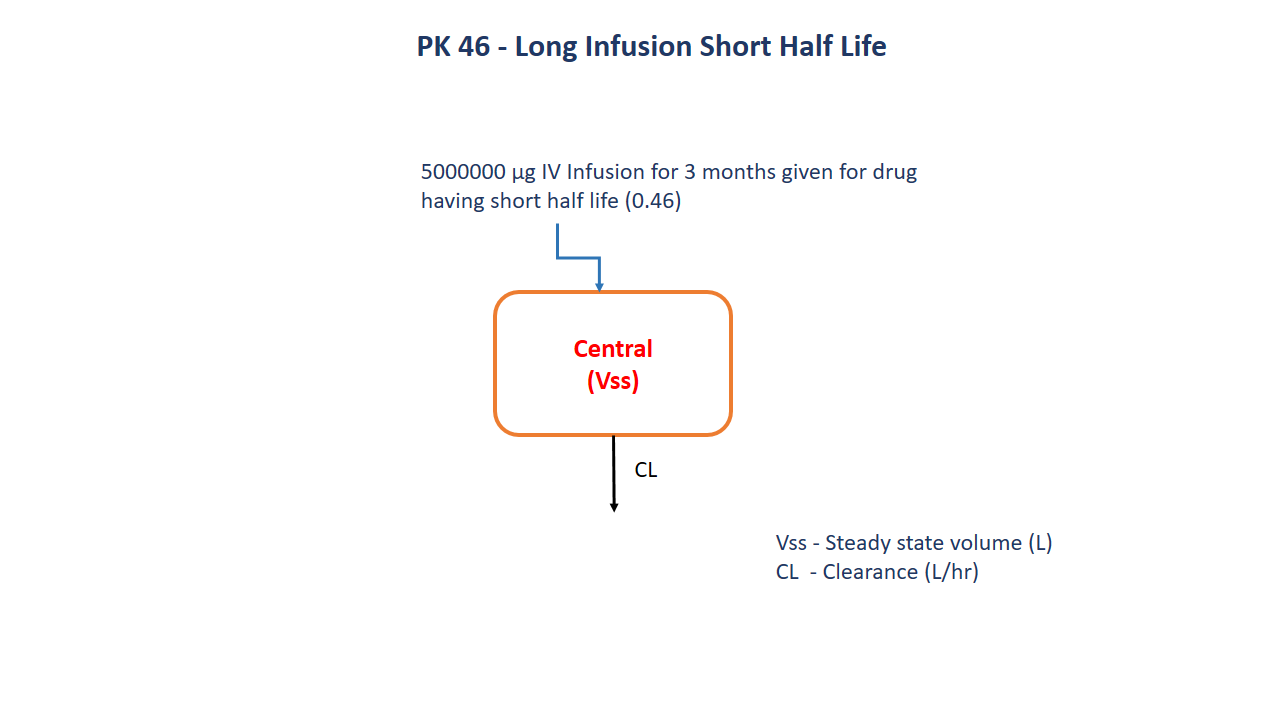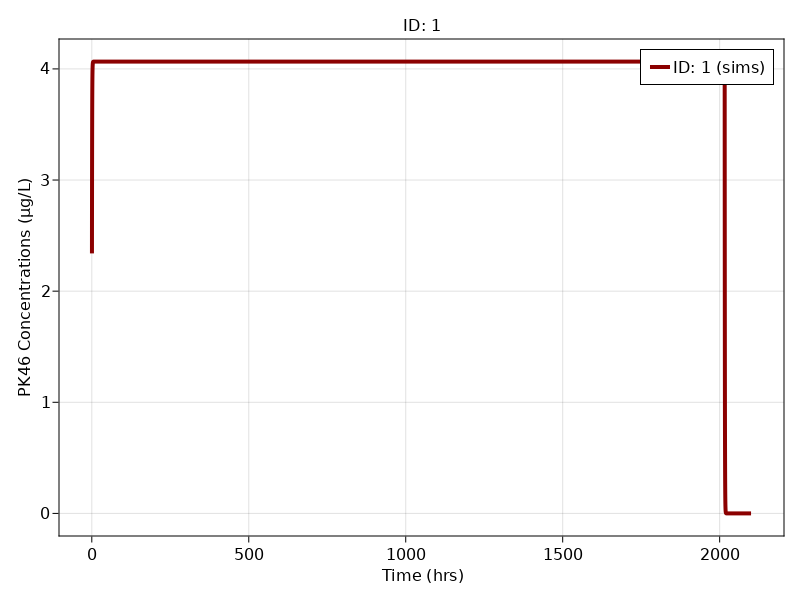# Exercise 46 - Long Infusion Short Half Life

### Background

• Structural model - Long infusion short half life one compartment model

• Route of administration - IV infusion

• Dosage Regimen - 500000 ug

• Number of Subjects - 1### Learning Outcome

This exercise deals with the drug having short half life.

### Objectives

To build a model for drug having short half life given as a very long infusion up to 3 months.

### Libraries

Call the "necessary" libraries to get started.

using Random
using Pumas
using PumasUtilities
using CairoMakie


### Model

To build a one compartment model for a drug having short half life given a long infusion for 3 months.

pk_46         = @model begin
desc    = "One Compartment Model"
timeu   = u"hr"
end

@param begin
tvvss   ∈ RealDomain(lower=0)
"Clearance (L/hr)"
tvcl    ∈ RealDomain(lower=0)
Ω       ∈ PDiagDomain(2)
end

@random begin
η       ~ MvNormal(Ω)
end

@pre begin
Vss     = tvvss*exp(η)
Cl      = tvcl*exp(η)
end

@dynamics begin
Central' =  - (Cl/Vss)*Central
end

@derived begin
cp       = @. Central/Vss
"""
Observed Concentration (ug/L)
"""
end
end

PumasModel
Random effects: η
Covariates:
Dynamical variables: Central
Derived: cp, dv
Observed: cp, dv


### Parameters

Parameters provided for simulation are as below. tv represents the typical value for parameters.

• $Vss$ - Steady state volume (L)

• $CL$ - Clearance (L/hr)

param = ( tvvss  = 35.6,
tvcl   = 61,
Ω      = Diagonal([0.0,0.0]),

(tvvss = 35.6, tvcl = 61, Ω = [0.0 0.0; 0.0 0.0], σ_add = 0.196337)


### DosageRegimen

A dose of 500000 ug was given as an Intravenous Infusion to a single subject for over 3 months.

ev1  = DosageRegimen(500000, time = 0, cmt = 1, duration = 2016)
sub1 = Subject(id = 1, events = ev1)

Subject
ID: 1
Events: 2


### Simulation

Simulate the data after the administration of Infusion

Random.seed!(123)
sim_sub1 = simobs(pk_46, sub1, param, obstimes = 0.5:0.1:2100)


### Visualization

f, a, p = sim_plot(pk_46, [sim_sub1],
observations = :cp,
color = :redsblues,
linewidth = 4,
axis = (xlabel = "Time (hrs)",
ylabel = "PK46 Concentrations (μg/L)",
xticks = 0:500:2000))
axislegend(a)
f